By taking a regular image as the input noise texture and the extracted gradients as the vector field (Figure 1), the image can be blurred (Figure 2) through a LIC process. Image warping (Figure 3) may be achieved when a separate vector field is used as the external driving force.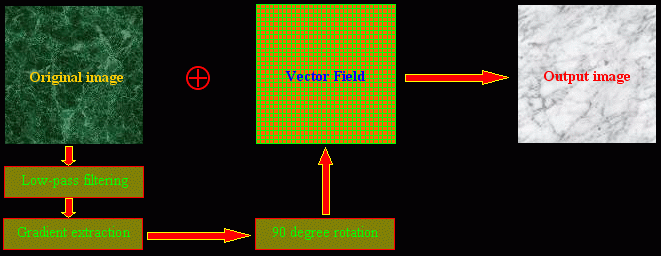Figure 1. Derive a vector field from the image itself to blur the image using LIC.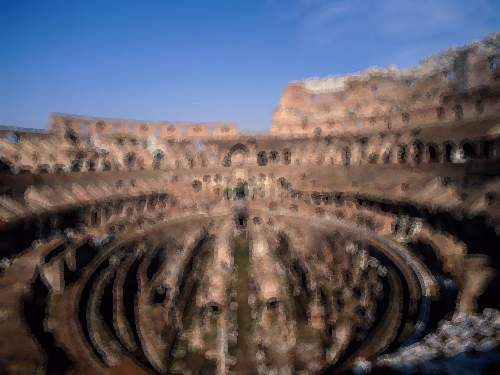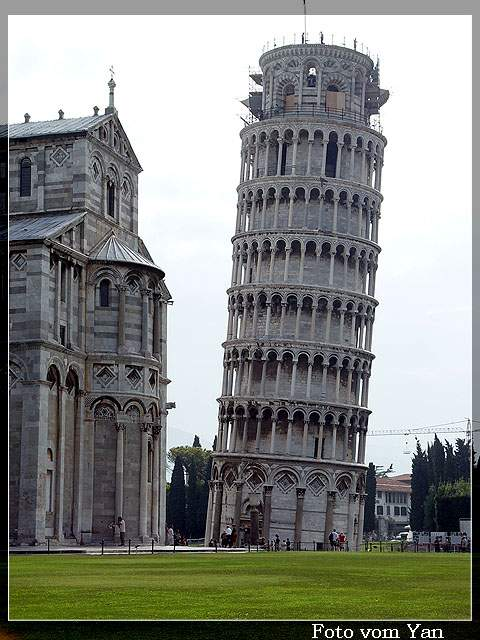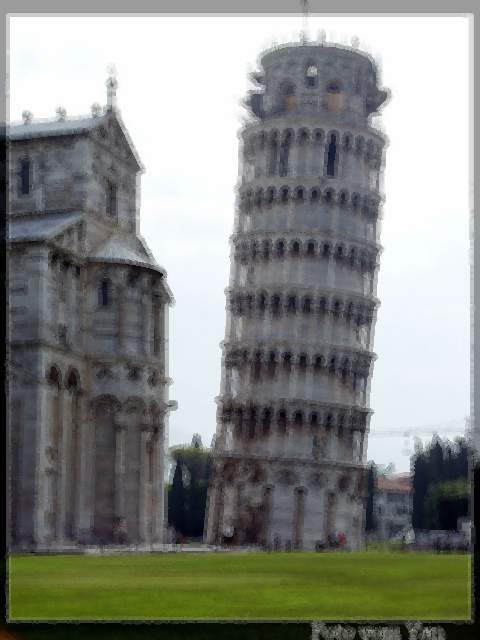Figure 2. Two images and the blurred results.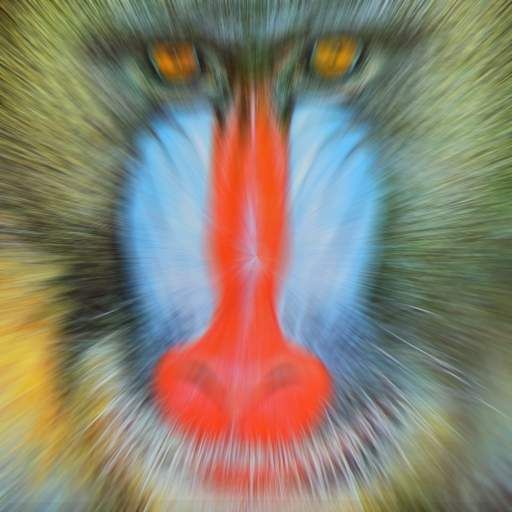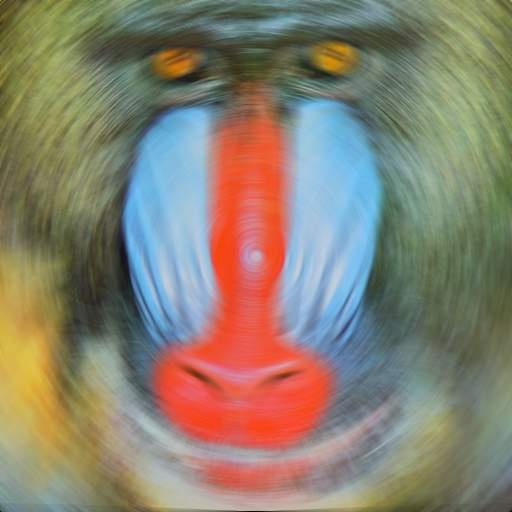Figure 3. Image warping.
 Line Integral Convolution Flow Visualization Research